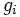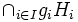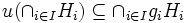# Nonempty intersection of cosets is coset of intersection

## Contents

DIRECT: The fact or result stated in this article has a trivial/direct/straightforward proof provided we use the correct definitions of the terms involved
View other results with direct proofs
VIEW FACTS USING THIS: directly | directly or indirectly, upto two steps | directly or indirectly, upto three steps|

## Statement

### Verbal statement

If the intersection of a collection of left cosets of subgroups is nonempty, then it is a coset of the intersection of the corresponding subgroups.

### Statement with symbols

Suppose$\{H_{i}\}_{i \in I}$ is a family of subgroups of a group$G$ indexed by$I$, and$g_{i}$ are elements of$G$. Then$\cap_{i \in I}g_{i}H_{i}$, if non-empty, is a left coset of the subgroup$\cap_{i \in I}H_{i}$.

## Proof

Given:$H_{i} \le G$,$g_{i}\in G$,$\cap_{i \in I}g_{i}H_{i}$ non-empty.

To prove: there exists$a \in G$ such that$\cap_{i \in I}g_{i}H_{i}=a\cap_{i \in I}H_{i}$

Proof: Observe that for any$u$ in$\cap_{i \in I}g_{i}H_{i}$, we have$g_{i}^{-1}u \in H_{i}$, viz:$u^{-1}g_{i} \in H_{i}$. So,$u^{-1}g_{i}H_{i}=H_{i}$.

For any$v \in \cap_{i \in I}H_{i}$, in each$H_{i}$ we can find$h_{i}$ such that$v=u^{-1}g_{i}h_{i}$. Therefore$uv$ is in$g_{i}H_{i}$. Hence$uv$ is in$\cap_{i \in I} g_{i}H_{i}$, viz:$u(\cap_{i \in I}H_{i}) \subseteq \cap_{i \in I} g_{i}H_{i}$.

Now, for all$u, p$ in$\cap_{i \in I}g_{i}H_{i}$, we can find$h_{i}, k_{i} \in H_{i}$ such that$u=g_{i}h_{i}$ and$p=g_{i}k_{i}$. Then$p^{-1}u=k_{i}^{-1}g_{i}^{-1}g_{i}h_{i}=k_{i}^{-1}h_{i} \in H_{i}$. So it follows that the cosets of the intersection subgroup with respect to$u, p$ are the same. Therefore,$\cap_{i \in I} g_{i}H_{i} \subseteq u(\cap_{i \in I}H_{i})$. Hence$\cap_{i \in I} g_{i}H_{i} = u(\cap_{i \in I}H_{i})$.